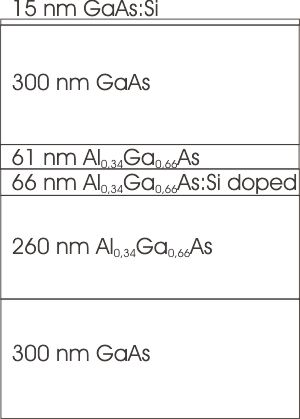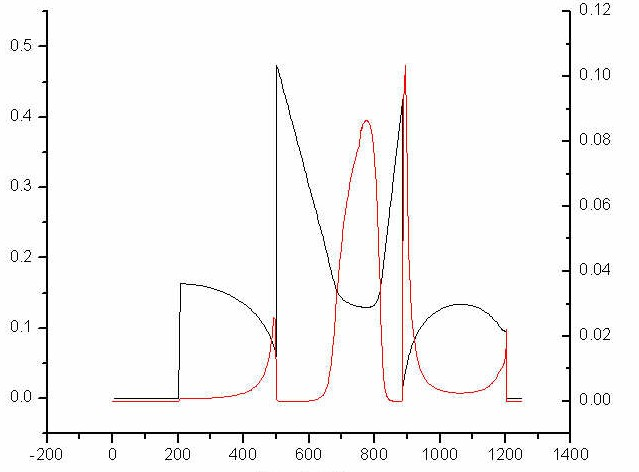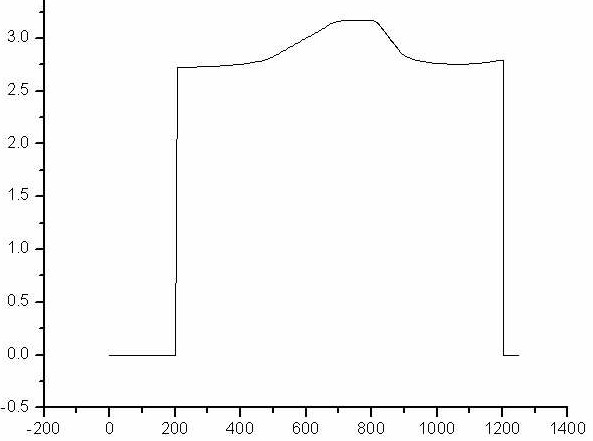nextnano.com  GUI: nextnanomat  Tool: nextnano++  Tool: nextnano³  Tool: nextnano.NEGF  Download | Search | Copyright | News | Publications  * password protected nextnano³ software1D inverted HEMT

# nextnano3 - Tutorial

## GaAs / AlGaAs  -  inverted High Electron Mobility Transistor (HEMT)

last updated: 13-03-19

Author: Stefan Birner

## GaAs / AlGaAs  -  inverted High Electron Mobility Transistor (HEMT)

• Here is the input file:``` invertedHEMT.in```
1. Step 7: GaAs / AlGaAs  -  inverted High Electron Mobility Transistor (HEMT) - MBE doped
2. The sample is 1137 nm, pseudomorphically grown on GaAs.
The interesting area is between 400 nm and 900 nm.
3. Again, we perform a one-dimensional simulation.
4. Just a reminder: If you need additional information about the keywords and their specifiers, you can look it up here.
5. The quantum region is over the whole device.
6. The inverted HEMT device looks like this:

_________________________________________________________________
1             2            3               4                5           6            7           8
GaAs       GaAs    AlGaAs   AlGaAs:Si   AlGaAs    GaAs   GaAs:Si   metal
200 nm       300        260            66               61        300         15          50
_________________________________________________________________
ohmic contact7. AlxGa1-xAs
We choose x to be 0.34.
In principle, we have a short period lattice (SPS)
SPS GaAs/AlAs (2 nm / 1 nm)
but we consider it due to the short period to be stoichiometric equivalent to Al0.34Ga0.66As which should simplify our calculations.
It improves the thermic resistence of the structures which underly a thermic annealing step for activation of the doping materials.
8. The flow scheme is 2:
1. calculate nonlinear Poisson as specified in input
(2. calculate current as specified in input)
For this example, we don't calculate the current.
9. Output
- The band structure will be saved into the directory``` band_structure/ ```- The densities will be saved into``` densities/ ```- The strain will be saved into``` strain1/ ```- Raw data will be saved into``` raw_data/ ```- kp data will be saved into` Schroedinger_kp/`
10. We are interested in plotting conduction band 1 and the resulting electron density to visualize the two 2DEGs (two dimensional electron gas) which should look somewhat like this:The black curve shows conduction band 1 and the red curve shows the electron density. Clearly, one can see that there are two triangular "bags" in the conduction band at GaAs/AlGaAs interfaces. If these bags lie below the Fermi energy, 2DEGs (two dimensional electron gases) can be formed. By variation of the doping concentration one should be able to generate zero, one or two 2DEGs. By increasing the doping concentration one should observe an increase in the number of electrons in the left GaAs/AlGaAs interface, which is not desired. The task is to avoid this and to get an 2DEG on the right side by choosing an appropriate doping profile.
11. The 15 nm GaAs layer is doped with a constant n-type Si doping (2.0*1018 cm-3). The donor level of Si in GaAs lies 5.8 meV below the conduction band.

```\$doping-function  doping-function-number = 2             ! ```acts as separator```  impurity-number        = 1             ! ```properties of this impurity type have```                                         ! ```to be specified later```  doping-concentration   = 2d0           ! 2.0*10^18 cm^-3  only-region            = 1187d0 1202d0 ! ```actually, only boarders of a line,```                                         ! ```rectangle, cube are allowed``` \$end_doping-function \$impurity-parameters  impurity-number             = 2          ! ```number, 1 or 2 ... (impurity numbers labeled in doping-function)```  impurity-name               = Si-in-GaAs ! ```a name (for later use - planned to read parameters from data base)```  impurity-type               = n-type     ! n-type, p-type, trap  number-of-energy-levels     = 1          ! ```number of energy levels of this impurity```  energy-levels-relative      = 0.0058d0   ! ```energy relative to 'nearest' band edge```                                           ! (n-type -> ```conduction band, else valence band```)                                           ! ```Si in GaAs: 5.8 meV below conduction band```  degeneracy-of-energy-levels = 2          ! ```degeneracy of energy levels (`2 `for n-type, ``` 4 ```for p-type)``` \$end_impurity-parameters```
12. The interior doping looks like this (to be revised!!).
Experimental details: Si is implanted by FIB (focused ion beam) on an amorphous As layer which will be removed after the implantation process.```\$doping-function  doping-function-number     = 1  impurity-number            = 1  base-function-1            = gauss-1d                          ! ```a valid base function name```  apply-function-1-along-dir = 0 0 1                             ! (0 0 1) , (0 1 0) , (1 0 0)  parameters-base-function-1 = 792.677d0 71.064d0 0.0d0 16.955d0 ! ``` center-coordinate width minimum-value maximum-value```  doping-concentration       = 1.5d0                             ! ``` doping concentration refers to that position```  only-region                = 672d0 822d0  position                   = 792.677d0 \$end_doping-function \$impurity-parameters  impurity-number             = 1  impurity-name               = Si-in-GaAs  impurity-type               = n-type  number-of-energy-levels     = 1  energy-levels-relativ       = 0.0058d0  degeneracy-of-energy-levels = 2 \$end_impurity-parameters```
13. A plot of the potential looks like this:• Please help us to improve our tutorial. Please send comments to``` support [at] nextnano.com```.